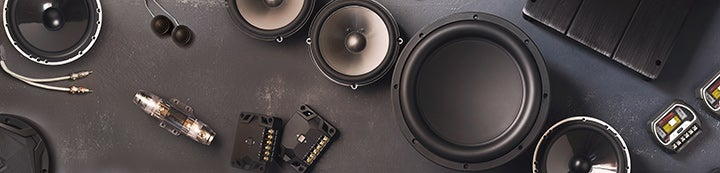1 - 6 of 6 Posts

Mrdeli100

·
Registered
Joined
·
7 Posts
Discussion Starter · ·
Hello at present I am tinkering around with speakers in my Audi Q5.

I understand that amp outputs at 4 ohms.

The front door speakers are 2 ohms and the dash tweeters (which are on the same channel) are 8 ohm with a 10uf capacitor.

I was intending to replace the front door speaker with a 4 ohm speaker and the dash tweeter with a 4 ohm speaker (I will replace the amp at a later date).

I have looked at charts and these would suggest that the 8 ohm tweeter is crossed over at around 1800 based on the fact it is 8 ohms.

This got me thinking......

As the amp outputs at 4 ohm, does the 10uf capacitor cut off the frequency at 4000 (as the amp is 4 ohm) or at 1800 (as the speaker is 8 ohm).

In a nutshell is the cut off set by the output of the amp or the ohms of the speaker?

Many thanks Mark

miniSQ

·
Registered
Joined
·
9,181 Posts
first off amps don't output at 4ohm. A better way to look at it is that amps produce a specified amount of power at various impedances (ohms).

Speakers are then built/designed to have a particular resistance (measured in ohms).

Then capacitors that you are referring to, they will block lower frequencies, and what frequencies they block will be determined by the resistance of the speaker. You can look up the charts online if you have already done so.

So the short answer to your question is, the speaker determines the XO point of the cap.

Holmz

·
Joined
·
7,487 Posts
first off amps don't output at 4ohm. A better way to look at it is that amps produce a specified amount of power at various impedances (ohms).

Speakers are then built/designed to have a particular resistance (measured in ohms).

Then capacitors that you are referring to, they will block lower frequencies, and what frequencies they block will be determined by the resistance of the speaker. You can look up the charts online if you have already done so.

So the short answer to your question is, the speaker determines the XO point of the cap.
Well said, another way of saying it is...
An amp outputs to try and hit a voltages hat is moving with the input signal.
An amp that is rated at 4-ohms can supply enough amperage to a 4-ohm load to drive some amount of watts.
An amp that is rated capable of delivering into a 2-ohm load will be delivering twice the amps (and hence twice the watts).

Mrdeli100

·
Registered
Joined
·
7 Posts
Discussion Starter · ·

gijoe

·
Registered
Joined
·
9,375 Posts
It's very simple, but people overlook what the words resistance and impedance mean. A speaker resists (makes it more difficult, if you will) the flow of electricity, how much it resists in measured in ohms and expressed either as resistance, or impedance. Both are essentially the same, but resistance refers to DC, and impedance to AC.

This is an oversimplification, but it's useful.

An amp outputs power. How much power is determined by how difficult the speaker makes it (impedance). Low impedance means the speaker doesn't make it that difficult, so the amp can easily send a lot of power. SO, as mentioned, the speaker determines the amps output. The frequency that a capacitor blocks is determined by the impedance, so an 8ohm tweeter and a 10uf cap would result in roughly a 2khz high pass. If you put a 4 ohm tweeter in it's place then the high pass will become 4khz.

1 - 6 of 6 Posts